Home > CC4 > Chapter A > Lesson A.1.4 > ProblemA-46

A-46.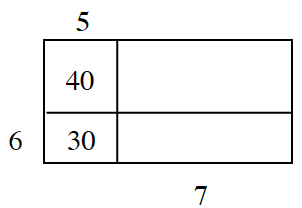Consider the rectangle at right. Homework Help ✎

1. Determine the perimeter of the large outer rectangle shown at right.

2. Notice that the areas of two of the parts have been labeled inside the rectangle. Calculate the total area. Remember to show all work leading to your solution.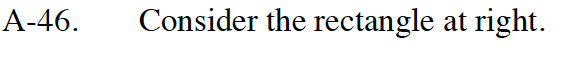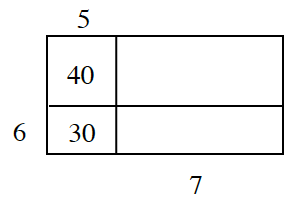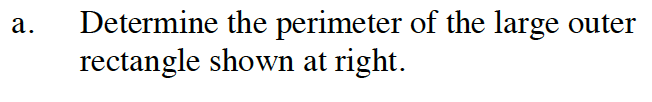You can find the missing side length using the area and side length given for the upper left inner rectangle.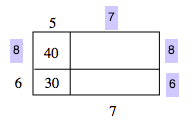52 units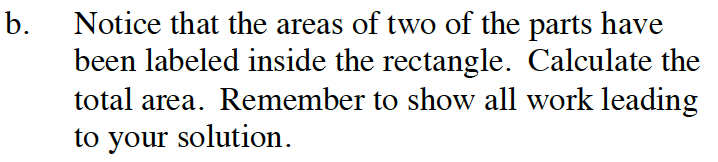168 square units